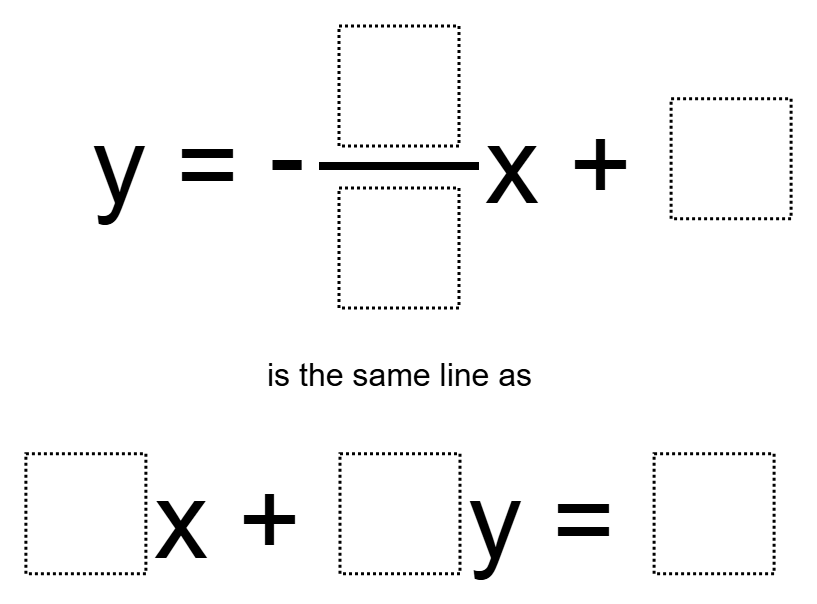Home > Grade 8 > Functions > Equivalent lines in slope-intercept and standard form

# Equivalent lines in slope-intercept and standard form

Directions: Using the digits 1 to 9 at most one time each, fill in the boxes to complete the statement below.### Hint

Which numbers are related in the two equations? How can we use this information to fill in the numbers that are “related”?

y=-3/1x+4 and 6x+2y=8

Source: Andy Schwen

## Scientific Notation 2

Directions: Using the digits 1 to 9 at most twice each, make the sum of …

1.2.•